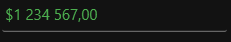# Appearance in WPF Currency TextBox

21 May 20216 minutes to read

This section deals with the appearance of `CurrencyTextBox` control and contains the following topics.

## Setting the Foreground

The CurrencyTextBox control Foreground can be modified based on the value of the control. The following are the foreground for `CurrencyTextBox` control.

### Foreground for Positive Value

We can change a positive color for the value of `CurrencyTextBox` by setting the PositiveForeground property and it will be applied when the Value is positive. The default color of `PositiveForeground` is `Black`.

``<syncfusion:CurrencyTextBox x:Name="currencyTextBox" Value="10" Width="100" Height="25" PositiveForeground="Blue" />``
``````CurrencyTextBox currencyTextBox = new CurrencyTextBox();
currencyTextBox.Width = 100;
currencyTextBox.Height = 25;
currencyTextBox.Value = 10;
currencyTextBox.PositiveForeground = Brushes.Blue;``````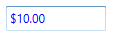### Foreground for Negative Value

We can change a negative color for the value of `CurrencyTextBox` by setting the NegativeForeground property and it will be applied when the ApplyNegativeForeground property is `true` and the `Value` is negative. The default color of `NegativeForeground` is `Red`.

``````<syncfusion:CurrencyTextBox x:Name="currencyTextBox" Value="-10" Width="100" Height="25"
NegativeForeground="SpringGreen" ApplyNegativeForeground="True" />``````
``````CurrencyTextBox currencyTextBox = new CurrencyTextBox();
currencyTextBox.Width = 100;
currencyTextBox.Height = 25;
currencyTextBox.Value = -10;
currencyTextBox.ApplyNegativeForeground = true;
currencyTextBox.NegativeForeground = Brushes.SpringGreen;``````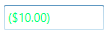### Foreground for Zero Value

We can change a zero color for the value of `CurrencyTextBox` by setting the ZeroColor property and it will be applied when the ApplyZeroColor property is `true` and the `Value` is zero.
The default color of `ZeroColor` is `Green`.

``````<syncfusion:CurrencyTextBox x:Name="currencyTextBox" Value="0" Width="100" Height="25"
ApplyZeroColor="True" ZeroColor="DarkGoldenrod"/>``````
``````CurrencyTextBox currencyTextBox = new CurrencyTextBox();
currencyTextBox.Width = 100;
currencyTextBox.Height = 25;
currencyTextBox.Value = 0;
currencyTextBox.ApplyZeroColor = true;
currencyTextBox.ZeroColor = Brushes.DarkGoldenrod;``````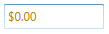## Setting the Background

`CurrencyTextBox` allows different brushes to fill the control. The Background property can be used to modify the control background color. The default color of `Background` is `White`.

``````<syncfusion:CurrencyTextBox x:Name="currencyTextBox" Width="100"
Height="25" Value ="80" Background="Cyan"/>``````
``````CurrencyTextBox currencyTextBox = new CurrencyTextBox();
currencyTextBox.Width = 100;
currencyTextBox.Height = 25;
currencyTextBox.Background = Brushes.Cyan;``````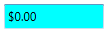Corner Radius indicates the degree to which the corners of the border can be rounded. To create curved borders for the `CurrencyTextBox`, use CornerRadius property. The default value of `CornerRadius` property is 1.

``<syncfusion:CurrencyTextBox x:Name="currencyTextBox" Width="100" Height="25" CornerRadius="5"/>``
``````CurrencyTextBox currencyTextBox = new CurrencyTextBox();
currencyTextBox.Width = 100;
currencyTextBox.Height = 25;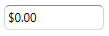## Apply Background for Selection

`CurrencyTextBox` allows different brushes to highlight the selected text by setting the SelectionBrush and SelectionOpacity properties. The `SelectionOpacity` property specifies the opacity of the `SelectionBrush`.

``<syncfusion:CurrencyTextBox x:Name="currencyTextBox" Width="100" Height="25" SelectionBrush="Red" SelectionOpacity="0.5"/>``
``````CurrencyTextBox currencyTextBox = new CurrencyTextBox();
currencyTextBox.Width = 100;
currencyTextBox.Height = 25;
currencyTextBox.SelectionBrush = Brushes.Red;
currencyTextBox.SelectionOpacity = 0.3;``````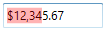## Align Value

`CurrencyTextBox` allows to display the value from right or center or left side by setting the TextAlignment property to `Right` or `Left` or `Center`. The Default value of `TextAlignment` is `Left`.

``<syncfusion:CurrencyTextBox x:Name="currencyTextBox" Width="100" Height="25" TextAlignment="Center"/>``
``````CurrencyTextBox currencyTextBox = new CurrencyTextBox();
currencyTextBox.Width = 100;
currencyTextBox.Height = 25;
currencyTextBox.TextAlignment = TextAlignment.Center;``````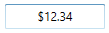## Setting ToolTip

`CurrencyTextBox` provides support for ToolTip to display certain information when the mouse hovers on the `CurrencyTextBox`. You can customize the tooltip information by setting the ToolTip property.

``<syncfusion:CurrencyTextBox x:Name="currencyTextBox" Width="100" Height="25" ToolTip="Enter Currency Value"/>``
``````CurrencyTextBox currencyTextBox = new CurrencyTextBox();
currencyTextBox.Width = 100;
currencyTextBox.Height = 25;
currencyTextBox.ToolTip = "Enter Currency Value";``````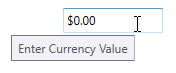## Theme

CurrencyTextBox supports various built-in themes. Refer to the below links to apply themes for the CurrencyTextBox,